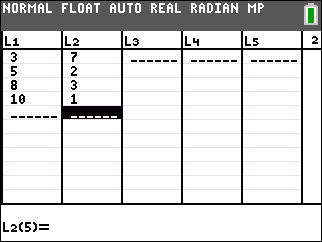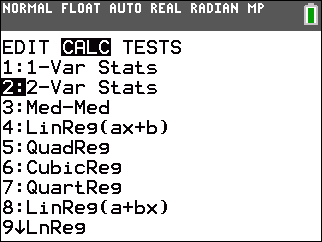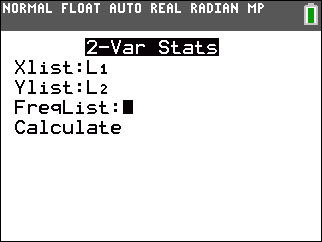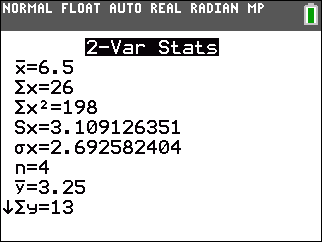# Knowledge Base

## Solution 34925: Calculating Two-Variable Statistics on the TI-84 Plus Family of Graphing Calculators.

### How do I calculate two-variable statistics on the TI-84 Plus family of graphing calculators?

Follow the example below to calculate two-variable statistics:

Data for this example:

L1: {3,5,8,10}
L2: {7,2,3,1}

• Press [STAT]  to access the STAT list editor.
• Enter the data in the L1 and L2 lists, making sure to press [ENTER] after each entry.Calculating the two-variable statistics:

• Press [STAT] [►]to highlight the CALC menu and press  to select the 2-Var Stats function.• From here, the XList should be L1, the YList should be L2 and the FreqList should be blank. You can press [2ND] [L1] to obtain L1, and then [2ND] [L2] to obtain L2 if necessary.• Press [ENTER] on "Calculate", and the two-variable statistics data will be displayed. The [▲] and [▼] keys can be used to scroll through the data.